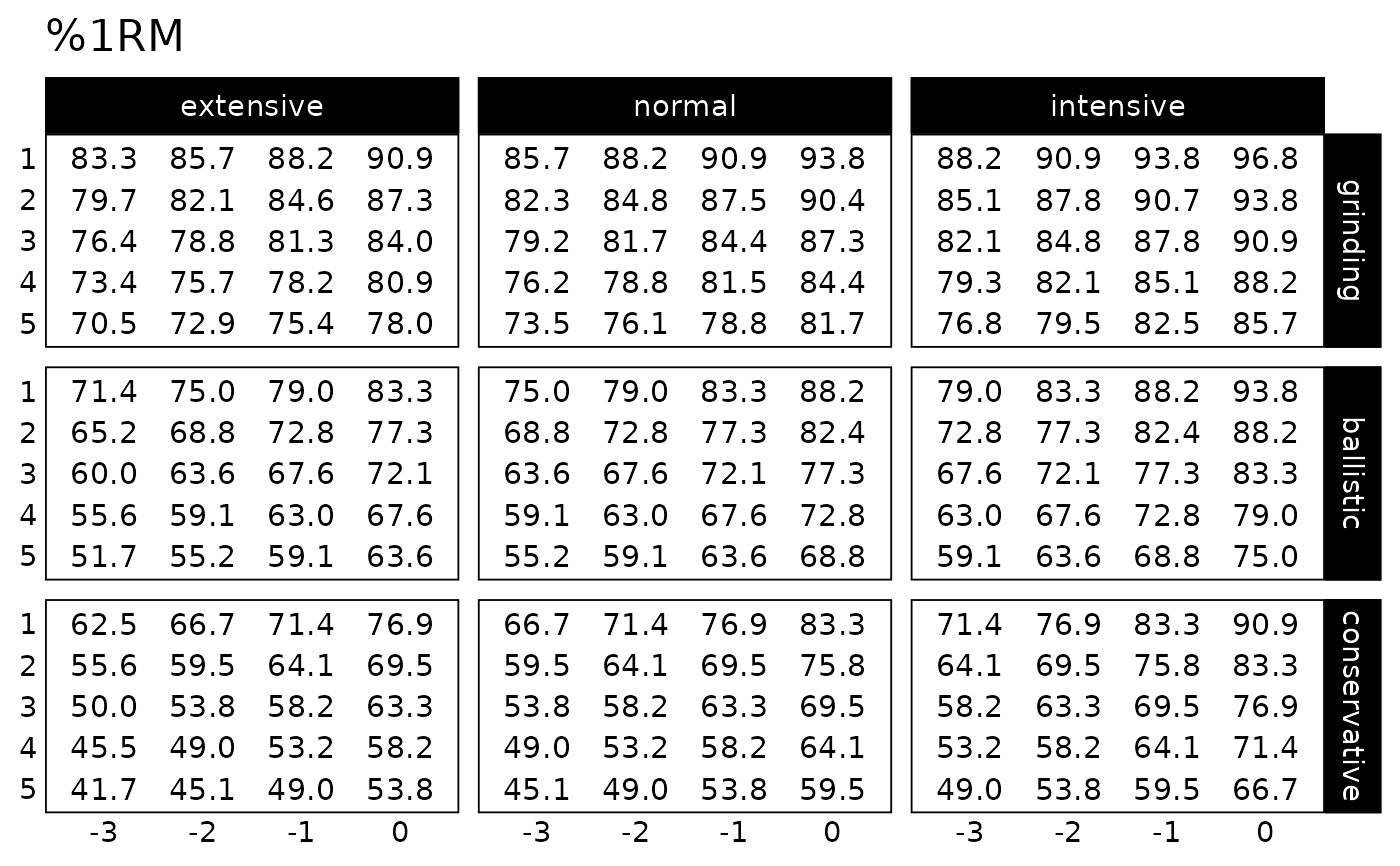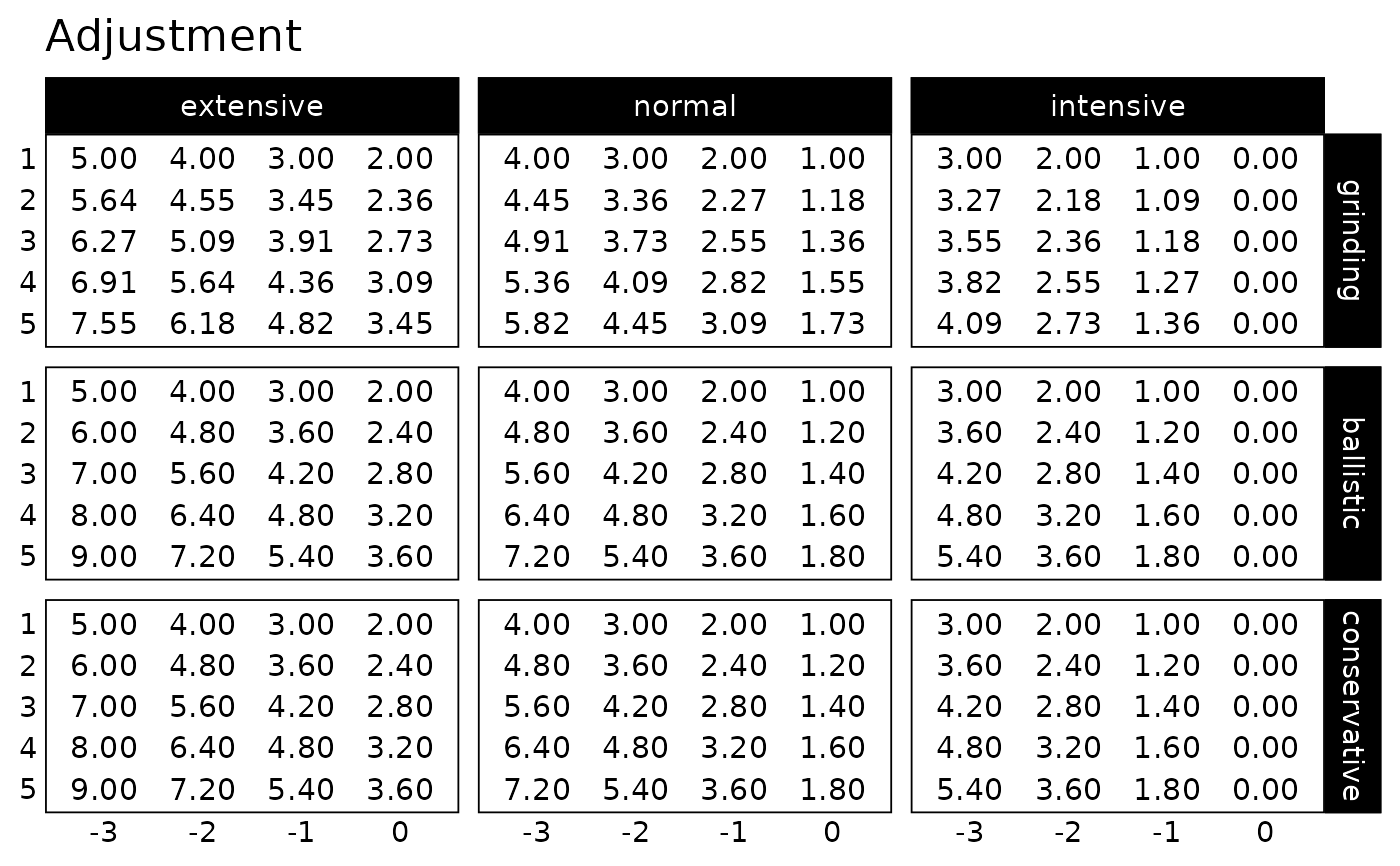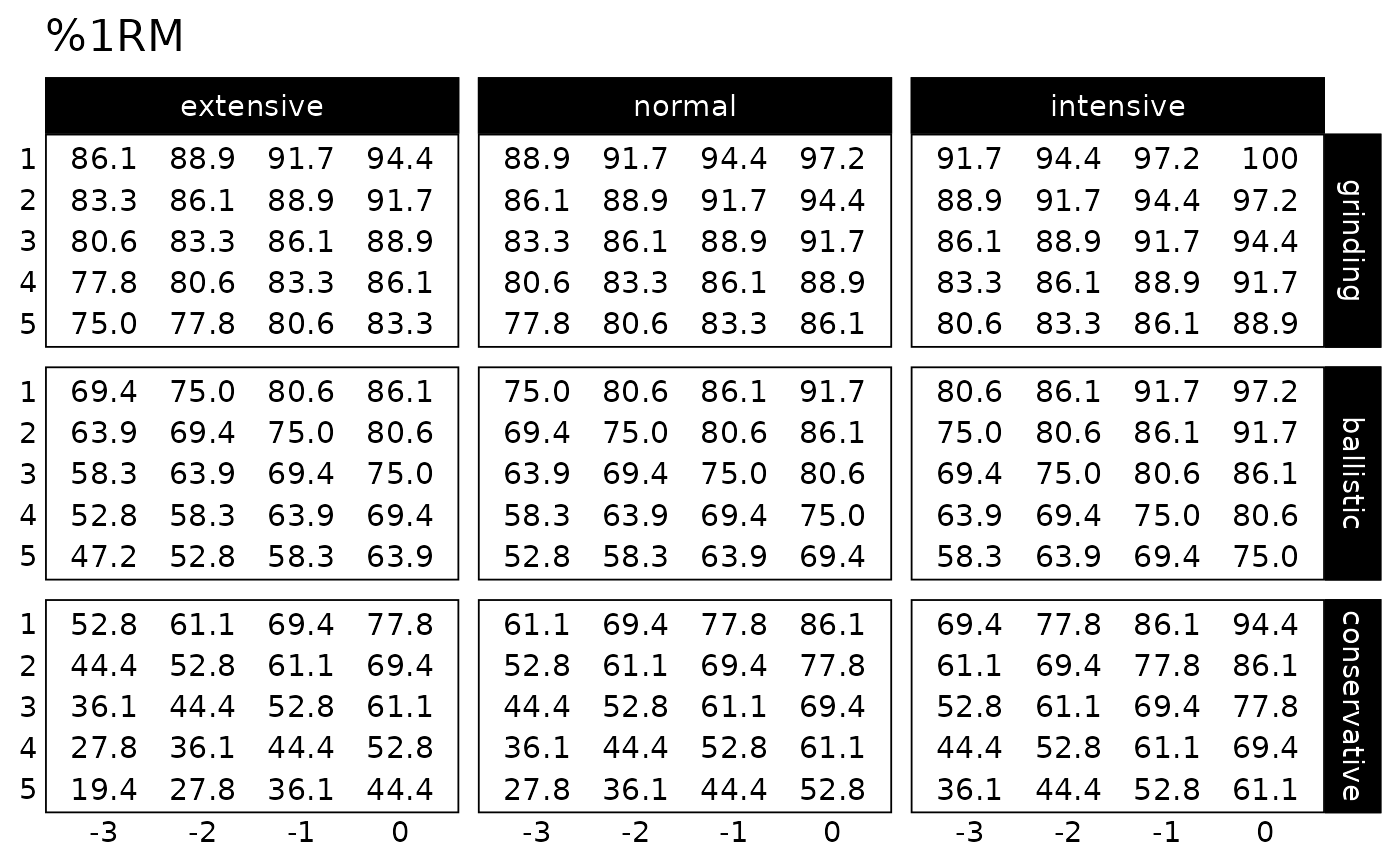Function for creating ggplot2 plot of the Progression Table

## Usage

plot_progression_table(
progression_table,
plot = "%1RM",
signif_digits = 3,
font_size = 14,
...
)

## Arguments

progression_table

Function for creating progression table

plot

Character string. Options include "%1RM" (default) and "adjustment"

signif_digits

Rounding numbers for plotting. Default is 3

Factor to multiply the adjustment. Useful when converting to percentage. Default is 1

font_size

Numeric. Default is 14

...

Forwarded to the generate_progression_table function

## Value

ggplot2 object

## Examples

plot_progression_table(progression_RIR_increment, "%1RM", reps = 1:5)plot_progression_table(progression_RIR_increment, "adjustment", reps = 1:5)# Create progression pot by using specific reps-max table and klin value
plot_progression_table(
progression_RIR,
reps = 1:5,
max_perc_1RM_func = max_perc_1RM_linear,
klin = 36
)